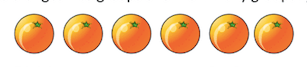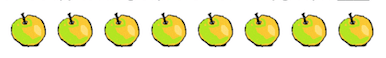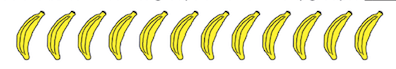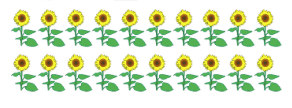Write times sentence 1
Write times sentence 2
Equal Groups
CHALLENGE
100

1 + 1 + 1 + 1 + 1 =

100

Write the multiplication sentence for:

2 + 2 + 2

2 x 3 or 2 x 3

100

Write the multiplication sentence for:3 x 3

100

There are 6 oranges. Circle groups of 2. How many groups are there?100

Write the multiplication sentence for:

6 + 6 + 6

6 x 3

200

2 + 2 + 2 + 2 + 2 =

10

200

Write the multiplication sentence for:

4 + 4 + 4 =

4 x 3 or 3 x 4
200

Write the multiplication sentence for:4 x 2 or 2 x 4

200

There are 8 apples. Circle groups of 4. How many groups are there?200

If I have 2 cups, and I put 3 pencils in each cup, how many pencils do I have in total?

300

3 + 3 + 3 + 3 =

12

300

Write the multiplication sentence for:

5 + 5 + 5 + 5 + 5

5 x 5

300

Write the multiplication sentence for:2 x 5 or 5 x 2

300

There are 9 nectarines. Circle groups of 3. How many groups are there?3

300

Write the multiplication sentence and tell me the answer

5 + 5 + 5 + 5 =

5 x 4 = 20

400

6 + 6 + 6 + 6 =

24

400

Write the multiplication sentence for:

7 + 7 + 7 + 7 + 7 =

7 x 5 or 5 x 7

400

Write the multiplication sentence for:3 x 5 or 5 x 3

400

There are 12 bananas. Circle groups of 3. How many groups are there?4

400

Kelly has 4 boxes. If she puts 5 teddy bears in each box, how many teddy bears does she have in total?

20

500

8 + 8 + 8 + 8 + 8 =

40

500

Write the multiplication sentence for:

9 + 9 + 9 + 9 + 9 + 9 + 9 + 9

9 x 8 or 8 x 9

500

Write the multiplication sentence for:4 x 5 or 5 x 4

500

There are 20 sunflowers. Circle groups of 4. How many groups are there?5

500

( 5 x 6 ) + 7 = ?

37

Click to zoom